Image Processing Toolbox User's GuideFiltering Using imfilter

Filtering of images, either by correlation or convolution, can be performed using the toolbox function `imfilter`. This example filters an image with a 5-by-5 filter containing equal weights. Such a filter is often called an averaging filter.

• ```I = imread('coins.png');
h = ones(5,5) / 25;
I2 = imfilter(I,h);
imshow(I), title('Original Image');
figure, imshow(I2), title('Filtered Image')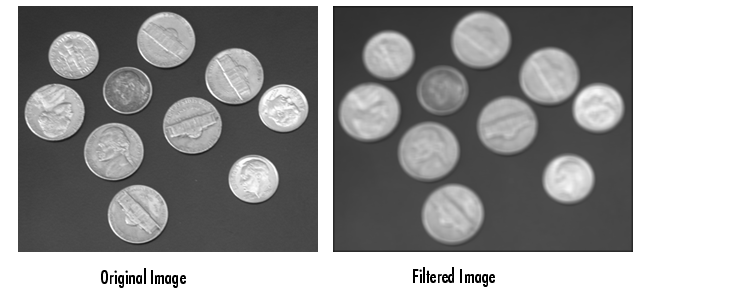```

Data Types

The `imfilter` function handles data types similarly to the way the image arithmetic functions do, as described in Image Arithmetic Saturation Rules. The output image has the same data type, or numeric class, as the input image. The `imfilter` function computes the value of each output pixel using double-precision, floating-point arithmetic. If the result exceeds the range of the data type, the `imfilter` function truncates the result to that data type's allowed range. If it is an integer data type, `imfilter` rounds fractional values.

Because of the truncation behavior, you might sometimes want to consider converting your image to a different data type before calling `imfilter`. In this example, the output of `imfilter` has negative values when the input is of class `double`.

• ```A = magic(5)

A =
17    24     1     8    15
23     5     7    14    16
4     6    13    20    22
10    12    19    21     3
11    18    25     2     9

h = [-1 0 1]

h =
-1     0     1

imfilter(A,h)

ans =
24   -16   -16    14    -8
5   -16     9     9   -14
6     9    14     9   -20
12     9     9   -16   -21
18    14   -16   -16    -2
```

Notice that the result has negative values. Now suppose `A` is of class `uint8`, instead of `double`.

• ```A = uint8(magic(5));
imfilter(A,h)

ans =

24     0     0    14     0
5     0     9     9     0
6     9    14     9     0
12     9     9     0     0
18    14     0     0     0
```

Since the input to `imfilter` is of class `uint8`, the output also is of class `uint8`, and so the negative values are truncated to `0`. In such cases, it might be appropriate to convert the image to another type, such as a signed integer type, `single`, or `double`, before calling `imfilter`.

Correlation and Convolution Options

The `imfilter` function can perform filtering using either correlation or convolution. It uses correlation by default, because the filter design functions, described in Filter Design, and the `fspecial` function, described in Using Predefined Filter Types, produce correlation kernels.

However, if you want to perform filtering using convolution instead, you can pass the string `'conv'` as an optional input argument to `imfilter`. For example:

• ```A = magic(5);
h = [-1 0 1]
imfilter(A,h)   % filter using correlation

ans =
24   -16   -16    14    -8
5   -16     9     9   -14
6     9    14     9   -20
12     9     9   -16   -21
18    14   -16   -16    -2

imfilter(A,h,'conv')   % filter using convolution

ans =

-24    16    16   -14     8
-5    16    -9    -9    14
-6    -9   -14    -9    20
-12    -9    -9    16    21
-18   -14    16    16     2
```

When computing an output pixel at the boundary of an image, a portion of the convolution or correlation kernel is usually off the edge of the image, as illustrated in the following figure.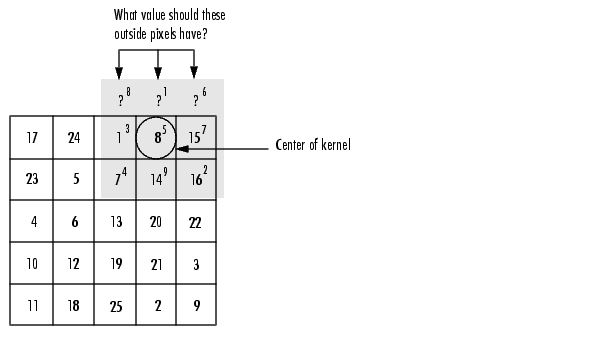When the Values of the Kernel Fall Outside the Image

The `imfilter` function normally fills in these off-the-edge image pixels by assuming that they are `0`. This is called zero padding and is illustrated in the following figure.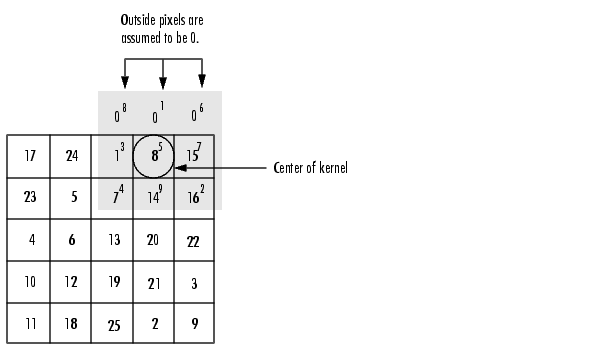When you filter an image, zero padding can result in a dark band around the edge of the image, as shown in this example.

• ```I = imread('eight.tif');
h = ones(5,5) / 25;
I2 = imfilter(I,h);
imshow(I), title('Original Image');
figure, imshow(I2), title('Filtered Image with Black Border')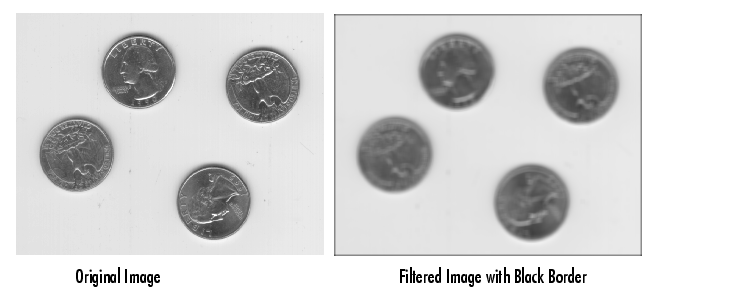```

To eliminate the zero-padding artifacts around the edge of the image, `imfilter` offers an alternative boundary padding method called border replication. In border replication, the value of any pixel outside the image is determined by replicating the value from the nearest border pixel. This is illustrated in the following figure.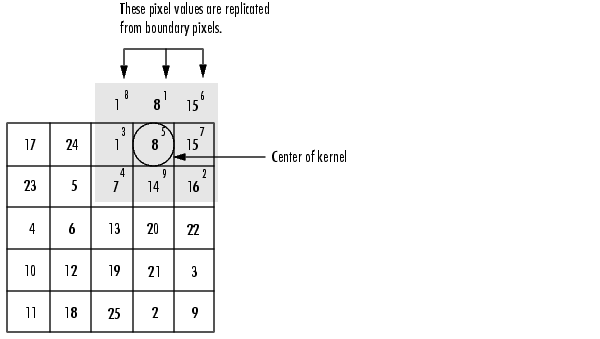Replicated Boundary Pixels

To filter using border replication, pass the additional optional argument `'replicate'` to `imfilter`.

• ```I3 = imfilter(I,h,'replicate');
figure, imshow(I3);
title('Filtered Image with Border Replication')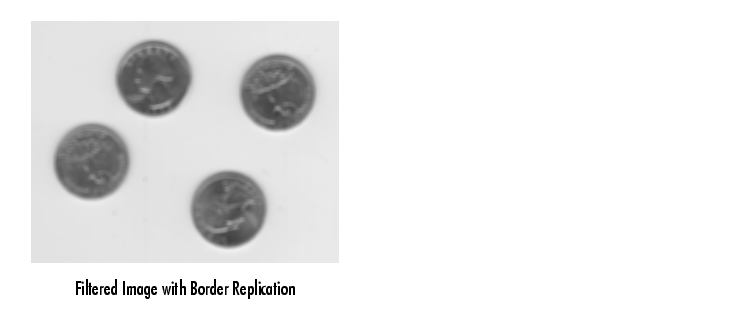```

The `imfilter` function supports other boundary padding options, such as `'circular'` and `'symmetric'`. See the reference page for `imfilter` for details.

Multidimensional Filtering

The `imfilter` function can handle both multidimensional images and multidimensional filters. A convenient property of filtering is that filtering a three-dimensional image with a two-dimensional filter is equivalent to filtering each plane of the three-dimensional image individually with the same two-dimensional filter. This example shows how easy it is to filter each color plane of a truecolor image with the same filter:

1. Read in an RGB image and display it.
• ```rgb = imread('peppers.png');
imshow(rgb);```
2. Filter the image and display it.
• ```h = ones(5,5)/25;
rgb2 = imfilter(rgb,h);
figure, imshow(rgb2)```

Relationship to Other Filtering Functions

MATLAB has several two-dimensional and multidimensional filtering functions. The function `filter2` performs two-dimensional correlation, `conv2` performs two-dimensional convolution, and `convn` performs multidimensional convolution. Each of these filtering functions always converts the input to `double`, and the output is always `double`. These other filtering functions always assume the input is zero padded, and they do not support other padding options.

In contrast, the `imfilter` function does not convert input images to `double`. The `imfilter` function also offers a flexible set of boundary padding options, as described in Boundary Padding Options.Correlation Using Predefined Filter Types